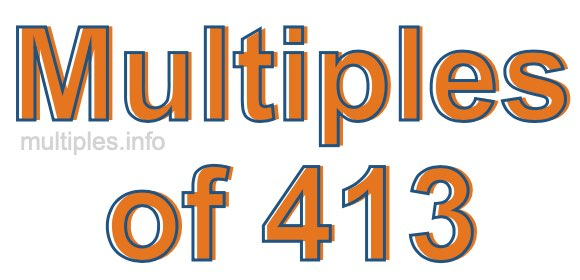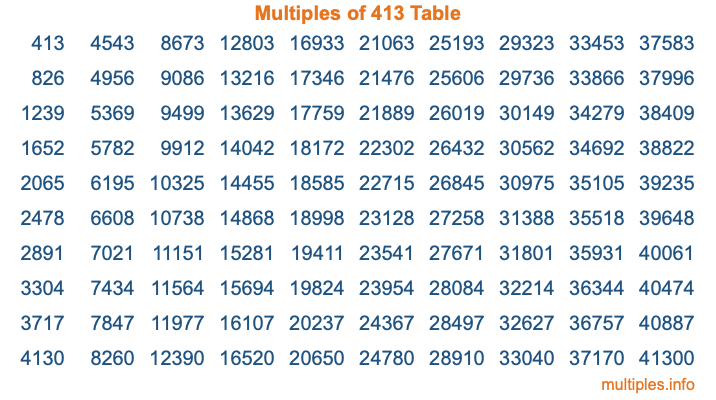Multiples of 413Welcome to the Multiples of 413 page. Here we will first teach you everything you will ever need to know about the multiples of 413, and then give you a study guide summary of everything we taught you to make sure you remember it all. Use this page to look up facts and learn information about the multiples of 413. This page will make you a multiples of four hundred thirteen expert!

Definition of Multiples of 413
Multiples of 413 are all the numbers that when divided by 413 equal an integer. Each of the multiples of 413 are called a multiple. A multiple of 413 is created by multiplying 413 by an integer.

Therefore, to create a list of multiples of 413, you start with 1 multiplied by 413, then 2 multiplied by 413, then 3 multiplied by 413, and so on for as long as you want. Thus, the list of the first five multiples of 413 is 413, 826, 1239, 1652, and 2065. To see a larger list of multiples of 413, see the printable image of Multiples of 413 further down on this page. We also have a category where you can choose any nth multiple of 413.

Multiples of 413 Checker
The Multiples of 413 Checker below checks to see if any number of your choice is a multiple of 413. In other words, it checks to see if there is any number (integer) that when multiplied by 413 will equal your number. To do that, we divide your number by 413. If the the quotient is an integer, then your number is a multiple of 413.

Is  a multiple of 413?

Least Common Multiple of 413 and ...
A Least Common Multiple (LCM) is the lowest multiple that two or more numbers have in common. This is also called the smallest common multiple or lowest common multiple and is useful to know when you are adding our subtracting fractions. Enter one or more numbers below (413 is already entered) to find the LCM.

Check out our LCM Calculator if you need more details about the Least Common Multiple or if you need the LCM for different numbers for adding and subtraction fractions.

nth Multiple of 413
As we stated above, 413 is the first multiple of 413, 826 is the second multiple of 413, 1239 is the third multiple of 413, and so on. Enter a number below to find the nth multiple of 413.

th multiple of 413

Multiples of 413 vs Factors of 413
413 is a multiple of 413 and a factor of 413, but that is where the similarities end. All postive multiples of 413 are 413 or greater than 413. All positive factors of 413 are 413 or less than 413.

Below is the beginning list of multiples of 413 and the factors of 413 so you can compare:

Multiples of 413: 413, 826, 1239, 1652, 2065, etc.

Factors of 413: 1, 7, 59, 413

As you can see, the multiples of 413 are all the numbers that you can divide by 413 to get a whole number. The factors of 413, on the other hand, are all the whole numbers that you can multiply by another whole number to get 413.

It's also interesting to note that if a number (x) is a factor of 413, then 413 will also be a multiple of that number (x).

Multiples of 413 vs Divisors of 413
The divisors of 413 are all the integers that 413 can be divided by evenly. Below is a list of the divisors of 413.

Divisors of 413: 1, 7, 59, 413

The interesting thing to note here is that if you take any multiple of 413 and divide it by a divisor of 413, you will see that the quotient is an integer.

Multiples of 413 Table
Below is an image of the first 100 multiples of 413 in a table. The table is in chronological order, column by column. The first column has the first ten multiples of 413, the second column has the next ten multiples of 413, and so on.The Multiples of 413 Table is also referred to as the 413 Times Table or Times Table of 413. You are welcome to print out our table for your studies.

Negative Multiples of 413
Although not often discussed or needed in math, it is worth mentioning that you can make a list of negative multiples of 413 by multiplying 413 by -1, then by -2, then by -3, and so on, to get the following list of negative multiples of 413:

-413, -826, -1239, -1652, -2065, etc.

Multiples of 413 Summary
Below is a summary of important Multiples of 413 facts that we have discussed on this page. To retain the knowledge on this page, we recommend that you read through the summary and explain to yourself or a study partner why they hold true.

There are an infinite number of multiples of 413.

A multiple of 413 divided by 413 will equal a whole number.

413 divided by a factor of 413 equals a divisor of 413.

The nth multiple of 413 is n times 413.

The largest factor of 413 is equal to the first positive multiple of 413.

413 is a multiple of every factor of 413.

413 is a multiple of 413.

A multiple of 413 divided by a divisor of 413 equals an integer.

413 divided by a divisor of 413 equals a factor of 413.

Any integer times 413 will equal a multiple of 413.

Multiples of a Number
Here you can get the multiples of another number, all with the same attention to detail as we did for multiples of 413 on this page.

Multiples of
Multiples of 414
Did you find our page about multiples of four hundred thirteen educational? Do you want more knowledge? Check out the multiples of the next number on our list!